#0
1
01. 云栖社区>
2. CDA数据分析师>
3. 博客>
4. 正文

## 教程 | 仅需六步，从零实现机器学习算法！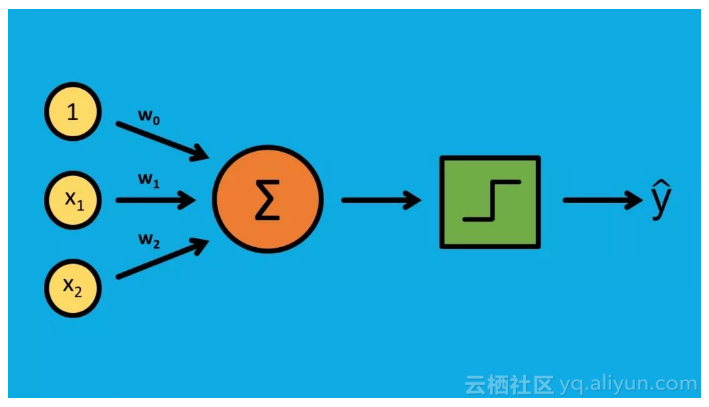●  对算法有基本的了解
●  找到不同的学习资源
●  将算法分解成块
●  从简单的例子开始
●  用可信的实现进行验证
●  写下你的过程

●  它是什么？
●  它一般用在什么地方？
●  什么时候不能用它？

●  单层感知器是最基础的神经网络，一般用于二分类问题（1 或 0，「是」或「否」）。
●  它可以应用在一些简单的地方，比如情感分析（积极反应或消极反应）、贷款违约预测（「会违约」，「不会违约」）。在这两种情况中，决策边界都是线性的。

●  当决策边界是非线性的时候不能使用感知器，要用不同的方法。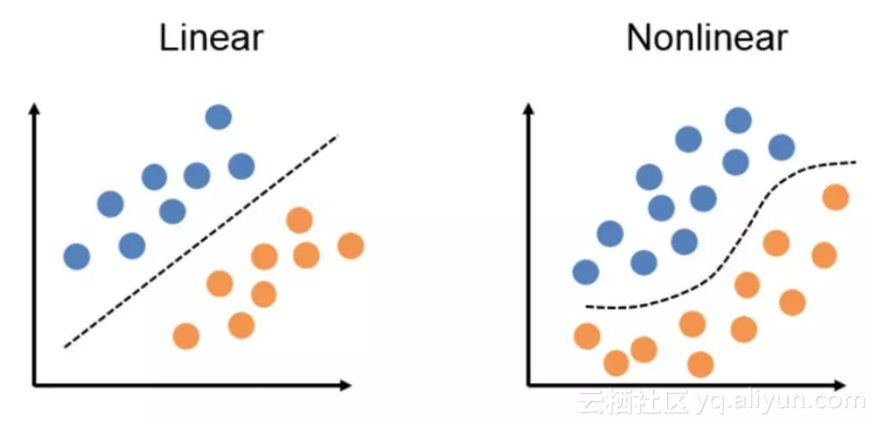●  《统计学习基础》（The Elements of Statistical Learning），第 4.5.1 节（https://web.stanford.edu/~hastie/Papers/ESLII.pdf）
●  《深入理解机器学习：从原理到算法》，第 21.4 节（https://www.cs.huji.ac.il/~shais/UnderstandingMachineLearning/understanding-machine-learning-theory-algorithms.pdf）

●  Jason Brownlee 写的《如何用 Python 从零开始实现感知器算法》（https://machinelearningmastery.com/implement-perceptron-algorithm-scratch-python/）
●  Sebastian Raschka 写的《单层神经网络和梯度下降》（https://sebastianraschka.com/Articles/2015_singlelayer_neurons.html）

●  初始化权重
●  将输入和权重相乘之后再求和
●  比较上述结果和阈值，计算输出（1 或 0）
●  更新权重
●  重复

1. 初始化权重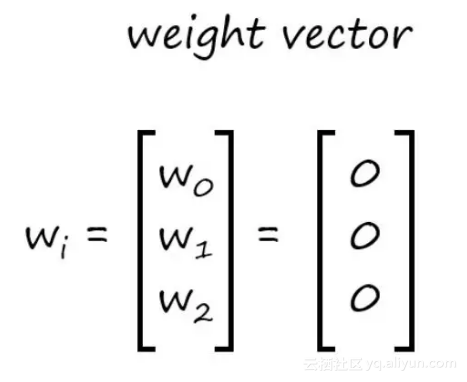2. 输入和权重相乘再求和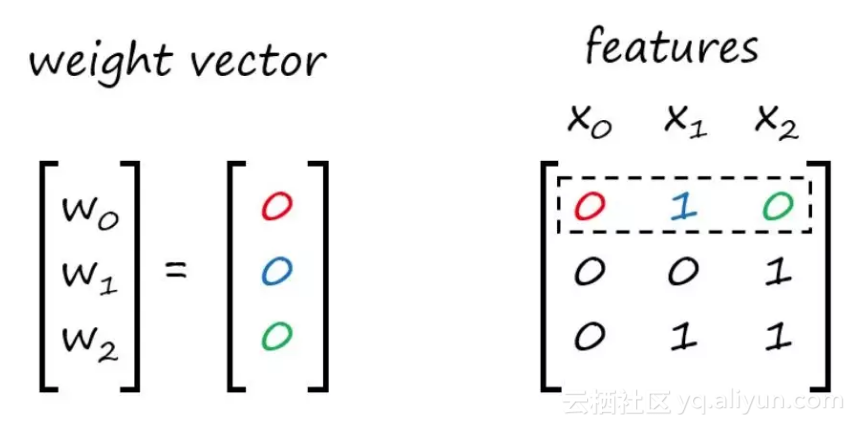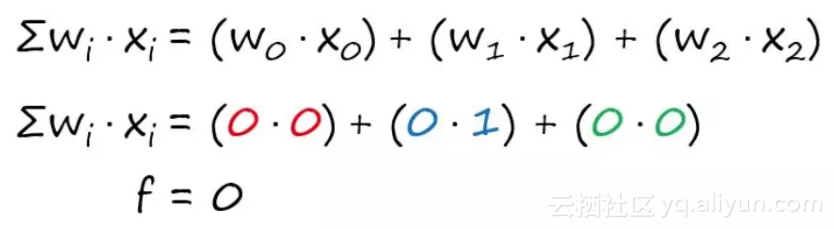3. 和阈值比较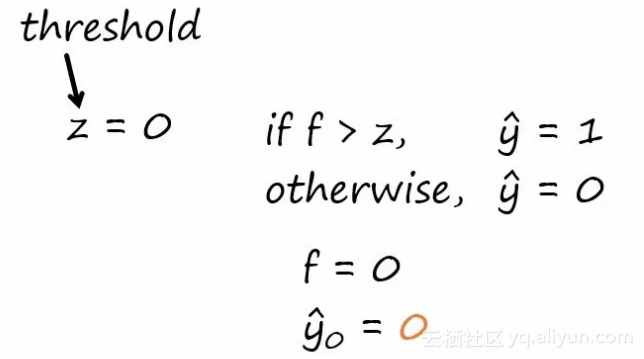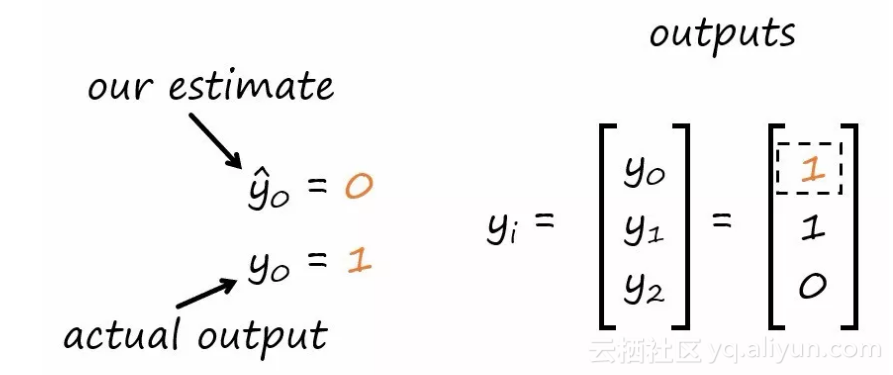4. 更新权重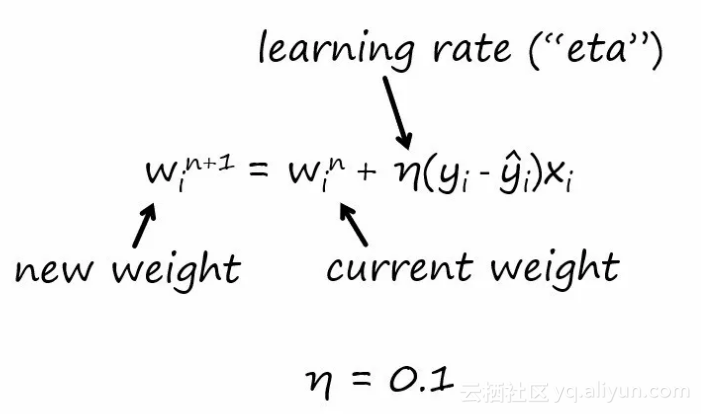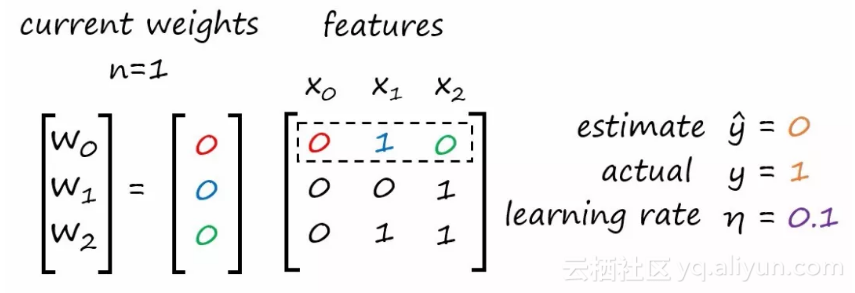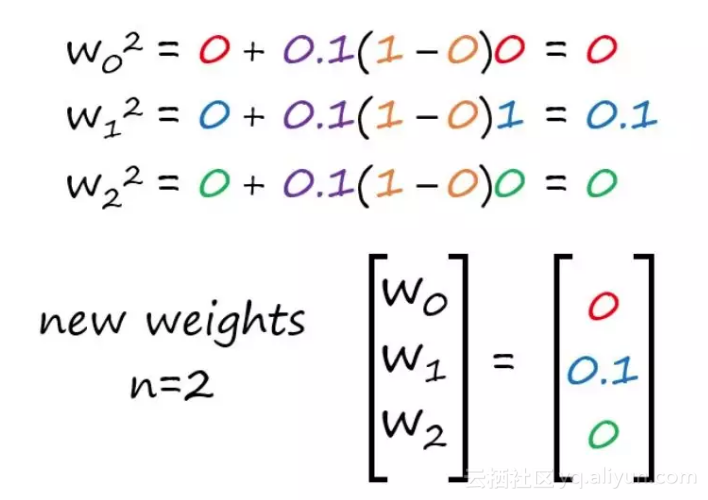5. 重复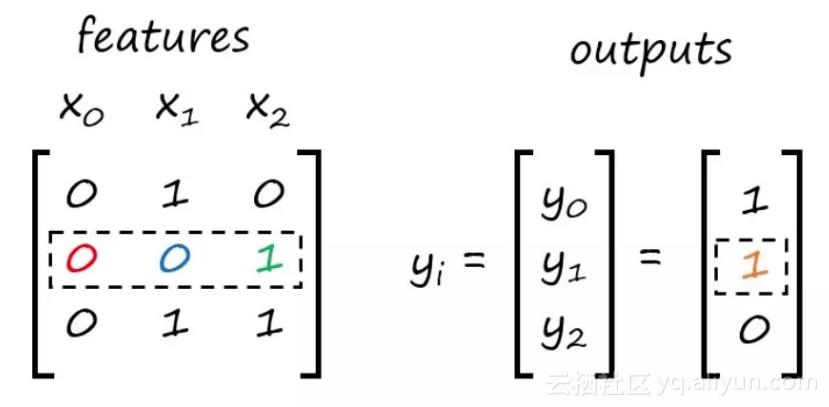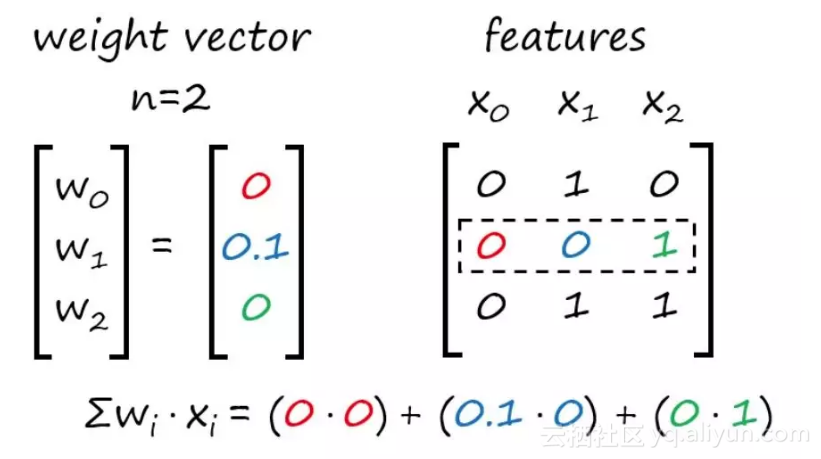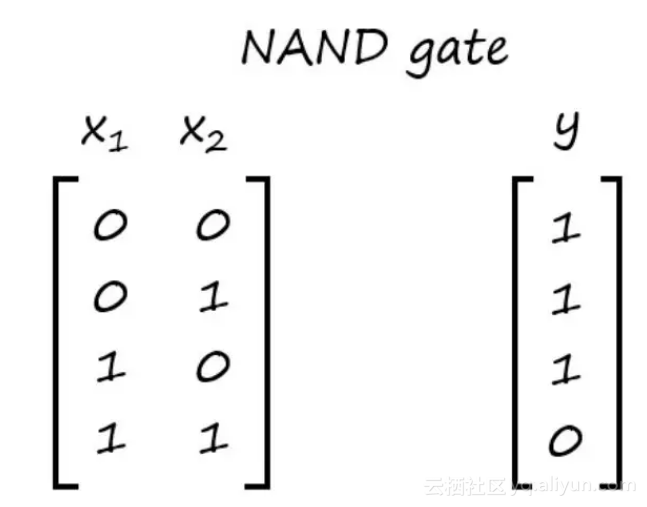``````
``````
`# Importing libraries`
``` ```
`# NAND Gate`
``` ```
`# Note: x0 is a dummy variable for the bias term`
``` ```
`# x0 x1 x2`
``` ```
`x = [[1., 0., 0.],`
``` ```
` [1., 0., 1.],`
``` ```
` [1., 1., 0.],`
``` ```
` [1., 1., 1.]]`
``` ```

``` ```
`y =[1.,`
``` ```
` 1.,`
``` ```
` 1.,`
``` ```
` 0.]`
``` ```

1. 初始化权重

``````
``````
`# Initialize the weights`
``` ```
`import numpy as np`
``` ```
`w = np.zeros(len(x))`
``` ```
``````
``````
`Out:`
``` ```
`[ 0. 0. 0.]`
``` ```

2. 将权重和输入相乘并对其求和

``````
``````
`# Dot Product`
``` ```
`f = np.dot(w, x)`
``` ```
`print f`
``` ```
``````
``````
`Out:`
``` ```
`0.0`
``` ```

3. 与阈值相比较

``````
``````
`# Activation Function`
``` ```
`z = 0.0`
``` ```
`if f > z:`
``` ```
` yhat = 1.`
``` ```
`else:`
``` ```
` yhat = 0.`
``` ```

``` ```
`print yhat`
``` ```
``````
``````
`Out:`
``` ```
`0.0`
``` ```

4. 更新权重

``````
``````
`# Update the weights`
``` ```
`eta = 0.1`
``` ```
`w = w + eta*(y - yhat)*x`
``` ```
`w = w + eta*(y - yhat)*x`
``` ```
`w = w + eta*(y - yhat)*x`
``` ```

``` ```
`print w`
``` ```
``````
``````
`Out:`
``` ```
`[ 0.1 0. 0. ]`
``` ```

5. 重复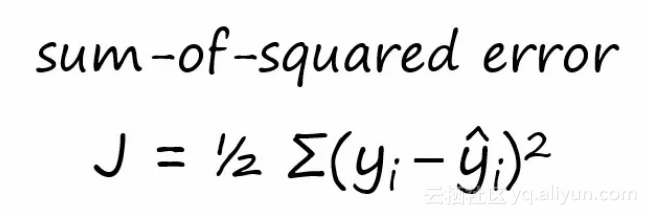``````
``````
`import numpy as np`
``` ```

``` ```

``` ```
`# Perceptron function`
``` ```
`def perceptron(x, y, z, eta, t):`
``` ```
` '''`
``` ```
` Input Parameters:`
``` ```
` x: data set of input features`
``` ```
` y: actual outputs`
``` ```
` z: activation function threshold`
``` ```
` eta: learning rate`
``` ```
` t: number of iterations`
``` ```
` '''`
``` ```

``` ```
` # initializing the weights`
``` ```
` w = np.zeros(len(x)) `
``` ```
` n = 0 `
``` ```

``` ```
` # initializing additional parameters to compute sum-of-squared errors`
``` ```
` yhat_vec = np.ones(len(y)) # vector for predictions`
``` ```
` errors = np.ones(len(y)) # vector for errors (actual - predictions)`
``` ```
` J = [] # vector for the SSE cost function`
``` ```

``` ```
` while n < t: for i in xrange(0, len(x)): # dot product f = np.dot(x[i], w) # activation function if f >= z: `
``` ```
` yhat = 1. `
``` ```
` else: `
``` ```
` yhat = 0.`
``` ```
` yhat_vec[i] = yhat`
``` ```

``` ```
` # updating the weights`
``` ```
` for j in xrange(0, len(w)): `
``` ```
` w[j] = w[j] + eta*(y[i]-yhat)*x[i][j]`
``` ```

``` ```
` n += 1`
``` ```
` # computing the sum-of-squared errors`
``` ```
` for i in xrange(0,len(y)): `
``` ```
` errors[i] = (y[i]-yhat_vec[i])**2`
``` ```
` J.append(0.5*np.sum(errors))`
``` ```

``` ```
` return w, J`
``` ```

``````
``````
`# x0 x1 x2`
``` ```
`x = [[1., 0., 0.],`
``` ```
` [1., 0., 1.],`
``` ```
` [1., 1., 0.],`
``` ```
` [1., 1., 1.]]`
``` ```

``` ```
`y =[1.,`
``` ```
` 1.,`
``` ```
` 1.,`
``` ```
` 0.]`
``` ```

``` ```
`z = 0.0`
``` ```
`eta = 0.1`
``` ```
`t = 50`
``` ```

``` ```
`print "The weights are:"`
``` ```
`print perceptron(x, y, z, eta, t)`
``` ```

``` ```
`print "The errors are:"`
``` ```
`print perceptron(x, y, z, eta, t)`
``` ```
``````
``````
`Out:`
``` ```
`The weights are:`
``` ```
`[ 0.2 -0.2 -0.1]`
``` ```
`The errors are:`
``` ```
`[0.5, 1.5, 1.5, 1.0, 0.5, 0.0, 0.0, 0.0, 0.0, 0.0, 0.0, 0.0, 0.0, 0.0, 0.0, 0.0, 0.0, 0.0, 0.0, 0.0, 0.0, 0.0, 0.0, 0.0, 0.0, 0.0, 0.0, 0.0, 0.0, 0.0, 0.0, 0.0, 0.0, 0.0, 0.0, 0.0, 0.0, 0.0, 0.0, 0.0, 0.0, 0.0, 0.0, 0.0, 0.0, 0.0, 0.0, 0.0, 0.0, 0.0]`
``` ```

●  导入数据
●  将数据分割为训练集和测试集
●  训练感知器
●  测试感知器
●  和 scikit-learn 感知器进行比较

1. 导入数据

``````
``````
`import pandas as pd`
``` ```
`import numpy as np`
``` ```
`import matplotlib.pyplot as plt`
``` ```

``` ```
`df = pd.read_csv("dataset.csv")`
``` ```
`plt.scatter(df.values[:,1], df.values[:,2], c = df['3'], alpha=0.8)`
``` ```

``` ```

``` ```
`text`
``` ```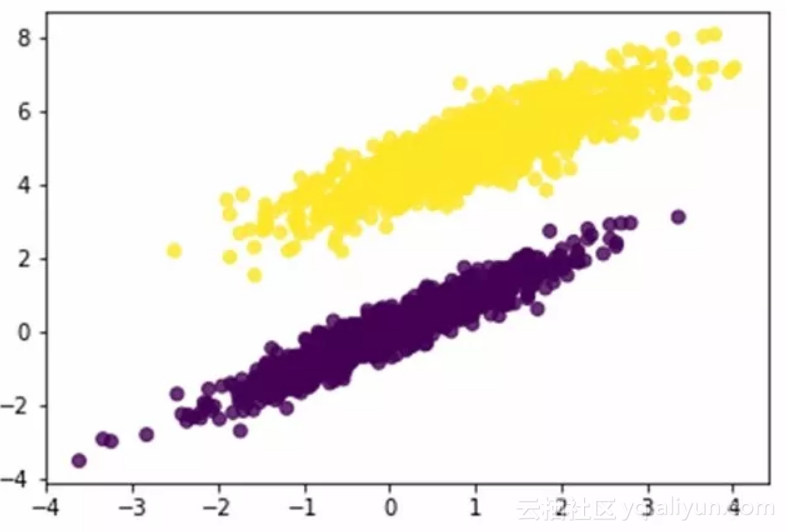2. 将数据分割成训练集/测试集

``````
``````
`df = df.values `
``` ```

``` ```
`np.random.seed(5)`
``` ```
`np.random.shuffle(df)`
``` ```

``````
``````
`train = df[0:int(0.7*len(df))]`
``` ```
`test = df[int(0.7*len(df)):int(len(df))]`
``` ```

``````
``````
`x_train = train[:, 0:3]`
``` ```
`y_train = train[:, 3]`
``` ```

``` ```
`x_test = test[:, 0:3]`
``` ```
`y_test = test[:, 3]`
``` ```

3. 训练感知器

``````
``````
`def perceptron_train(x, y, z, eta, t):`
``` ```
` '''`
``` ```
` Input Parameters:`
``` ```
` x: data set of input features`
``` ```
` y: actual outputs`
``` ```
` z: activation function threshold`
``` ```
` eta: learning rate`
``` ```
` t: number of iterations`
``` ```
` '''`
``` ```

``` ```
` # initializing the weights`
``` ```
` w = np.zeros(len(x)) `
``` ```
` n = 0 `
``` ```

``` ```
` # initializing additional parameters to compute sum-of-squared errors`
``` ```
` yhat_vec = np.ones(len(y)) # vector for predictions`
``` ```
` errors = np.ones(len(y)) # vector for errors (actual - predictions)`
``` ```
` J = [] # vector for the SSE cost function`
``` ```

``` ```
` while n < t: for i in xrange(0, len(x)): # dot product f = np.dot(x[i], w) # activation function if f >= z: `
``` ```
` yhat = 1. `
``` ```
` else: `
``` ```
` yhat = 0.`
``` ```
` yhat_vec[i] = yhat`
``` ```

``` ```
` # updating the weights`
``` ```
` for j in xrange(0, len(w)): `
``` ```
` w[j] = w[j] + eta*(y[i]-yhat)*x[i][j]`
``` ```

``` ```
` n += 1`
``` ```
` # computing the sum-of-squared errors`
``` ```
` for i in xrange(0,len(y)): `
``` ```
` errors[i] = (y[i]-yhat_vec[i])**2`
``` ```
` J.append(0.5*np.sum(errors))`
``` ```

``` ```
` return w, J`
``` ```

``` ```
`z = 0.0`
``` ```
`eta = 0.1`
``` ```
`t = 50`
``` ```

``` ```
`perceptron_train(x_train, y_train, z, eta, t)`
``` ```

``````
``````
`w = perceptron_train(x_train, y_train, z, eta, t)`
``` ```
`J = perceptron_train(x_train, y_train, z, eta, t)`
``` ```

``` ```
`print w`
``` ```
`print J`
``` ```
``````
``````
`Out:`
``` ```
`[-0.5 -0.29850122 0.35054929]`
``` ```
`[4.5, 0.0, 0.0, 0.0, 0.0, 0.0, 0.0, 0.0, 0.0, 0.0, 0.0, 0.0, 0.0, 0.0, 0.0, 0.0, 0.0, 0.0, 0.0, 0.0, 0.0, 0.0, 0.0, 0.0, 0.0, 0.0, 0.0, 0.0, 0.0, 0.0, 0.0, 0.0, 0.0, 0.0, 0.0, 0.0, 0.0, 0.0, 0.0, 0.0, 0.0, 0.0, 0.0, 0.0, 0.0, 0.0, 0.0, 0.0, 0.0, 0.0]`
``` ```

4. 测试感知器

``````
``````
`from sklearn.metrics import accuracy_score`
``` ```

``` ```
`w = perceptron_train(x_train, y_train, z, eta, t)`
``` ```

``` ```
`def perceptron_test(x, w, z, eta, t):`
``` ```
` y_pred = []`
``` ```
` for i in xrange(0, len(x-1)):`
``` ```
` f = np.dot(x[i], w) `
``` ```

``` ```
` # activation function`
``` ```
` if f > z: `
``` ```
` yhat = 1 `
``` ```
` else: `
``` ```
` yhat = 0`
``` ```
` y_pred.append(yhat)`
``` ```
` return y_pred`
``` ```

``` ```
`y_pred = perceptron_test(x_test, w, z, eta, t)`
``` ```

``` ```
`print "The accuracy score is:"`
``` ```
`print accuracy_score(y_test, y_pred)`
``` ```

5. 和 scikit-learn 感知器进行比较

``````
``````
`from sklearn.linear_model import Perceptron`
``` ```

``` ```
`# training the sklearn Perceptron`
``` ```
`clf = Perceptron(random_state=None, eta0=0.1, shuffle=False, fit_intercept=False)`
``` ```
`clf.fit(x_train, y_train)`
``` ```
`y_predict = clf.predict(x_test)`
``` ```

``````
``````
`Out:`
``` ```
`sklearn weights:`
``` ```
`[-0.5 -0.29850122 0.35054929]`
``` ```
`my perceptron weights:`
``` ```
`[-0.5 -0.29850122 0.35054929]`
``` ```

scikit-learn 模型中的权重和我们模型的权重完全相同。这意味着我们的模型可以正确地工作，这是个好消息。

●  你要更深刻地理解这个过程，因为你还要将你学到的东西教给别人。
●  你要向潜在雇主展示这个过程。

GitHub 个人资料是展示你所做工作的一种很好的方法。

+ 关注